# Guest post by Julien Mairal: A Kernel Point of View on Convolutional Neural Networks, part IIThis is a continuation of Julien Mairal‘s guest post on CNNs, see part I here.

Stability to deformations of convolutional neural networks

In their ICML paper Zhang et al. introduce a functional space for CNNs with one layer, by noticing that for some dot-product kernels, smoothed variants of rectified linear unit activation functions (ReLU) live in the corresponding RKHS, see also this paper and that one. By following a similar reasoning with multiple layers, it is then possible to show that the functional space described in part I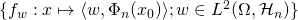contains CNNs with such smoothed ReLU, and that the norm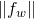of such networks can be controlled by the spectral norms of filter matrices. This is consistent with previous measures of complexity for CNNs, see this paper by Bartlett et al.

A perhaps more interesting finding is that the abstract representation, which only depends on the network architecture, may provide near-translation invariance and stability to small image deformations while preserving information—that is,can be recovered from. The original characterization we use was introduced by Mallat in his paper on the scattering transform—a multilayer architecture akin to CNNs based on wavelets, and was extended to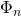by Alberto Bietti, who should be credited for all the hard work here.

Our goal is to understand under which conditions it is possible to obtain a representation that (i) is near-translation invariant, (ii) is stable to deformations, (iii) preserves signal information. Given a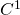-diffeomorphism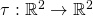and denoting by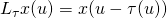its action operator (for an image defined on the continuous domain), the main stability bound we obtain is the following one, see Theorem 7 in Mallat’s paper if, for all,whereare universal constants,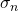is the scale parameter of the pooling operatorcorresponding to the “amount of pooling” performed up to the last layer,is the maximum pixel displacement and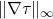represents the maximum amount of deformation, see the paper for the precise definitions of all these quantities. Note that when, the representationbecomes translation invariant: indeed, consider the particular case of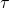being a translation, thenand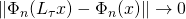.

The stability bound and a few additional results tell us a few things about the network architecture: (a) small patches lead to more stable representations (the dependency is hidden in); (b) signal preservation for discrete signals requires small subsampling factors (and thus small pooling) between layers. In such a setting, the scale parameterstill grows exponentially with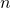and near translation invariance may be achieved with several layers.

Interestingly, we may now come back to the Cauchy-Schwarz inequality from part 1, and note that ifis stable, the RKHS normis then a natural quantity that provides stability to deformations to the prediction function, in addition to measuring model complexity in a traditional sense.

Feature learning in RKHSs and convolutional kernel networks

The previous paragraph is devoted to the characterization of convolutional architectures such as CNNs but the previous kernel construction can in fact be used to derive more traditional kernel methods. After all, why should one spend efforts defining a kernel between images if not to use it?

This can be achieved by considering finite-dimensional approximations of the previous feature maps. In order to shorten the presentation, we simply describe the main idea based on the Nystrom approximation and refer to the paper for more details. Approximating the infinite-dimensional feature maps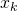(see the figure at the top of part I) can be done by projecting each point in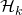onto a-dimensional subspace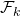leading to a finite-dimensional feature map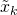akin to CNNs, see the figure at the top of the post.

By parametrizingwithanchor points, and using a dot-product kernel, a patchfrom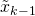is encoded through the mapping functionwhereis applied pointwise. Then, computingfromadmits a CNN interpretation, where only the normalization and the matrix multiplication by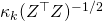are not standard operations. It remains now to choose the anchor points:

• kernel approximation: a first approach consists of using a variant of the Nystrom method, see this paper and that one. When plugging the corresponding image representation in a linear classifier, the resulting approach behaves as a classical kernel machine. Empirically, we observe that the higher the number of anchor points, the better the kernel approximation, and the higher the accuracy. For instance, a two-layer network with a-dimensional representations achieves aboutaccuracy on CIFAR-10 without data augmentation (see here).
• back-propagation, feature selection: learning the anchor pointscan also be done as in a traditional CNN, by optimizing them end-to-end. This allows using deeper lower-dimensional architectures and empirically seems to perform better when enough data is available, e.g.,accuracy on CIFAR-10 with simple data augmentation. There, the subspacesare not learned anymore to provide the best kernel approximation, but the model seems to perform a sort of feature selection in each layer’s RKHS, which is not well understood yet (This feature selection interpretation is due to my collaborator Laurent Jacob).

Note that the first CKN model published here was based on a different approximation principle, which was not compatible with end-to-end training. We found this to be less scalable and effective.

Other links between neural networks and kernel methods

Finally, other links between kernels and infinitely-wide neural networks with random weights are classical, but they were not the topic of this blog post (they should be the topic of another one!). In a nutshell, for a large collection of weights distributions and nonlinear functions, the following quantity admits an analytical formwhere the termsmay be seen as an infinitely-wide single-layer neural network. The first time such a relation appears is likely to be in the PhD thesis of Radford Neal with a Gaussian process interpretation, and it was revisited later by Le Roux and Bengio and by Cho and Saul with multilayer models.

In particular, whenis the rectified linear unit andfollows a Gaussian distribution, it is known that we recover the arc-cosine kernel. We may also note that random Fourier features also yield a similar interpretation.

Other important links have also been drawn recently between kernel regression and strongly over-parametrized neural networks, see this paper and that one, which is another exciting story.

This entry was posted in Machine learning. Bookmark the permalink.

## 2 Responses to "Guest post by Julien Mairal: A Kernel Point of View on Convolutional Neural Networks, part II"

••